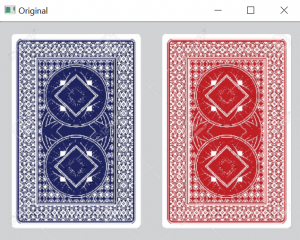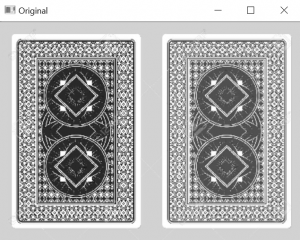Open In App
Related Articles

# OpenCV: Segmentation using Thresholding

But before moving into anymore detail, below is a brief overview of OpenCV.

OpenCV (Open Source Computer Vision) is a cross platform, open-source library of programming functions, aimed at performing real-time computer vision tasks in a wide variety of fields, such as:

• Facial recognition
• Iris Recognition Systems
• Gesture recognition
• Human–computer interaction (HCI)
• Mobile robotics
• Object identification
• Segmentation and recognition
• Stereopsis stereo vision: depth perception from 2 cameras
• Augmented reality

It also includes a robust statistical machine learning library, that contains a number of different classifiers used to support the above areas.

To use OpenCV, simply import or include the required libraries and start making use of the myriad of available functions.

Thresholding is a very popular segmentation technique, used for separating an object from its background. In the article below, I have described various techniques used to threshold grayscale images(8-bit).

The process of thresholding involves, comparing each pixel value of the image (pixel intensity) to a specified threshold. This divides all the pixels of the input image into 2 groups:

1. Pixels having intensity value lower than threshold.
2. Pixels having intensity value greater than threshold.

These 2 groups are now given different values, depending on various segmentation types.
OpenCV supports 5 different thresholding schemes on Grayscale(8-bit) images using the function :

Double threshold(InputArray src, OutputArray dst, double thresh, double maxval, int type)

Parameters:

• InputArray src: Input Image (Mat, 8-bit or 32-bit)
• OutputArray dst: Output Image ( same size as input)
• double thresh: Set threshold value
• double maxval: maxVal, used in type 1 and 2
• int type* :Specifies the type of threshold to be use. (0-4)
• *Below a list of thresholding types is given.

Input ImageThe input RGB image is first converted to a grayscale image before thresholding is done.Thresholding types

To compile OpenCV programs, you need to have OpenCV library installed on your system. I will be posting a simple tutorial for the same, in the coming days.
If you have already installed OpenCV, run the below code with the input image of your choice.

 `// CPP program to demonstrate segmentation``// thresholding.``#include ``#include ``#include ``#include `` ` `using` `namespace` `cv;`` ` `int` `main(``int` `argc, ``char``** argv)``{``    ``if` `(argc != 2) ``    ``{``        ``cout << ``" Usage: "``                ``" "` `<< endl;``        ``return` `-1;``    ``}`` ` `    ``int` `threshold_value = 0;`` ` `    ``// Valid Values: 0, 1, 2, 3, 4``    ``int` `threshold_type = 2; `` ` `    ``// maxVal, useful for threshold_type 1 and 2``    ``int` `maxVal = 255; `` ` `    ``// Source image``    ``Mat src = imread(argv, 1);`` ` `    ``cvNamedWindow(``"Original"``, CV_WINDOW_NORMAL);``    ``imshow(``"Original"``, src);`` ` `    ``Mat src_gray, dst;`` ` `    ``// Convert the image to GrayScale``    ``cvtColor(src, src_gray, CV_BGR2GRAY);`` ` `    ``// Create a window to display results``    ``cvNamedWindow(``"Result"``, CV_WINDOW_NORMAL);`` ` `    ``createTrackbar(``"Threshold"``, ``"Result"``, ``                  ``&threshold_value, 255);``    ``while` `(1) ``    ``{``        ``threshold(src_gray, dst, threshold_value, ``                         ``maxVal, threshold_type);``        ``imshow(``"Result"``, dst);``        ``waitKey(1);``    ``}``}`